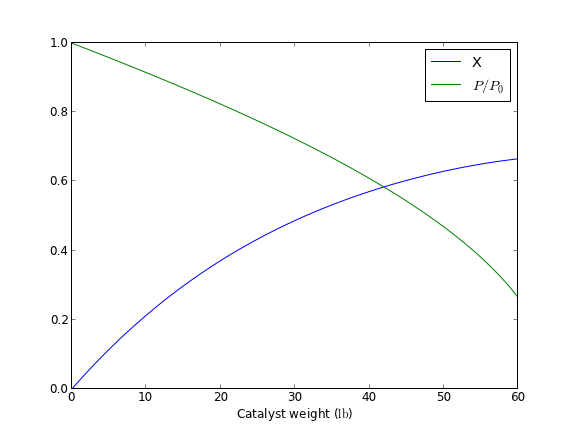## Using events in odelay with multiple equations

| categories: odes | tags: events | View Comments

odelay was recently updated to support multiple odes with events. Here is an example. We want the solutions to:

\begin{align} \frac{dy_1}{dx} = y_2 \\ \frac{dy_2}{dx} = -y_1 \end{align}

with initial conditions $$y_1(0) = 2$$ and $$y_2(0) = 1$$. We want to stop the integration when $$y_2 = -1$$ and find out when $$dy_1/dx=0$$ and at a maximum.

from pycse import odelay
import matplotlib.pyplot as plt
import numpy as np

def ode(Y,x):
y1, y2 = Y
dy1dx = y2
dy2dx = -y1
return [dy1dx, dy2dx]

def event1(Y, x):
y1, y2 = Y
value = y2 - (-1.0)
isterminal = True
direction  = 0
return value, isterminal, direction

def event2(Y, x):
dy1dx, dy2dx = ode(Y,x)
value = dy1dx - 0.0
isterminal = False
direction = -1  # derivative is decreasing towards a maximum
return value, isterminal, direction

Y0 = [2.0, 1.0]
xspan = np.linspace(0, 5)
X, Y, XE, YE, IE = odelay(ode, Y0, xspan, events=[event1, event2])

plt.plot(X, Y)
for ie,xe,ye in zip(IE, XE, YE):
if ie == 1: #this is the second event
y1,y2 = ye
plt.plot(xe, y1, 'ro')

plt.legend(['$y_1$', '$y_2$'], loc='best')
plt.savefig('images/odelay-mult-eq.png')
plt.show()


Here are the plotted results:org-mode source

## Units in ODEs

| categories: | tags: | View Comments

We reconsider a simple ODE but this time with units. We will use the quantities package again.

Here is the ODE, $$\frac{dCa}{dt} = -k Ca$$ with $$C_A(0) = 1.0$$ mol/L and $$k = 0.23$$ 1/s. Compute the concentration after 5 s.

import quantities as u

k = 0.23 / u.s
Ca0 = 1 * u.mol / u.L

return -k * Ca

import numpy as np
from scipy.integrate import odeint

tspan = np.linspace(0, 5) * u.s

print sol[-1]

[ 0.31663678]


No surprise, the units are lost. Now we start wrapping odeint. We wrap everything, and then test two examples including a single ODE, and a coupled set of ODEs with mixed units.

import quantities as u
import matplotlib.pyplot as plt

import numpy as np
from scipy.integrate import odeint as _odeint

def odeint(func, y0, t, args=(),
Dfun=None, col_deriv=0, full_output=0,
ml=None, mu=None, rtol=None, atol=None,
tcrit=None, h0=0.0, hmax=0.0, hmin=0.0,
ixpr=0, mxstep=0, mxhnil=0, mxordn=12,
mxords=5, printmessg=0):

def wrapped_func(Y0, T, *args):
# put units on T if they are on the original t
# check for units so we don't put them on twice
if not hasattr(T, 'units') and hasattr(t, 'units'):
T = T * t.units
# now for the dependent variable units. Y0 may be a scalar or
# a list or an array. we want to check each element of y0 for
# units, and add them to the corresponding element of Y0 if we
# need to.
try:
uY0 = [x for x in Y0] # a list copy of contents of Y0
# this works if y0 is an iterable, eg. a list or array
for i, yi in enumerate(y0):
if not hasattr(uY0[i],'units') and hasattr(yi, 'units'):

uY0[i] = uY0[i] * yi.units

except TypeError:
# we have a scalar
if not hasattr(Y0, 'units') and hasattr(y0, 'units'):
uY0 = Y0 * y0.units

val = func(uY0, t, *args)

try:
return np.array([float(x) for x in val])
except TypeError:
return float(val)

if full_output:
y, infodict = _odeint(wrapped_func, y0, t, args,
Dfun, col_deriv, full_output,
ml, mu, rtol, atol,
tcrit, h0, hmax, hmin,
ixpr, mxstep, mxhnil, mxordn,
mxords, printmessg)
else:
y = _odeint(wrapped_func, y0, t, args,
Dfun, col_deriv, full_output,
ml, mu, rtol, atol,
tcrit, h0, hmax, hmin,
ixpr, mxstep, mxhnil, mxordn,
mxords, printmessg)

# now we need to put units onto the solution units should be the
# same as y0. We cannot put mixed units in an array, so, we return a list
m,n = y.shape # y is an ndarray, so it has a shape
if n > 1: # more than one equation, we need a list
uY = [0 for yi in range(n)]

for i, yi in enumerate(y0):
if not hasattr(uY[i],'units') and hasattr(yi, 'units'):
uY[i] = y[:,i] * yi.units
else:
uY[i] = y[:,i]

else:
uY = y * y0.units

y = uY

if full_output:
return y, infodict
else:
return y

##################################################################
# test a single ODE
k = 0.23 / u.s
Ca0 = 1 * u.mol / u.L

return -k * Ca

tspan = np.linspace(0, 5) * u.s

print sol[-1]

plt.plot(tspan, sol)
plt.xlabel('Time ({0})'.format(tspan.dimensionality.latex))
plt.ylabel('$C_A$ ({0})'.format(sol.dimensionality.latex))
plt.savefig('images/ode-units-ca.png')

##################################################################
# test coupled ODEs
lbmol = 453.59237*u.mol

kprime = 0.0266 * lbmol / u.hr / u.lb
Fa0 = 1.08 * lbmol / u.hr
alpha = 0.0166 / u.lb
epsilon = -0.15

def dFdW(F, W, alpha0):
X, y = F
dXdW = kprime / Fa0 * (1.0 - X)/(1.0 + epsilon * X) * y
dydW = - alpha0 * (1.0 + epsilon * X) / (2.0 * y)
return [dXdW, dydW]

X0 = 0.0 * u.dimensionless
y0 = 1.0

# initial conditions
F0 = [X0, y0] # one without units, one with units, both are dimensionless

wspan = np.linspace(0,60) * u.lb

sol = odeint(dFdW, F0, wspan, args=(alpha,))
X, y = sol

print 'Test 2'
print X[-1]
print y[-1]

plt.figure()
plt.plot(wspan, X, wspan, y)
plt.legend(['X','$P/P_0$'])
plt.xlabel('Catalyst weight ({0})'.format(wspan.dimensionality.latex))
plt.savefig('images/ode-coupled-units-pdrpo.png')

[ 0.31663678] mol/L
Test 2
0.665569578156 dimensionless
0.263300470681That is not too bad. This is another example of a function you would want to save in a module for reuse. There is one bad feature of the wrapped odeint function, and that is that it changes the solution for coupled ODEs from an ndarray to a list. That is necessary because you apparently cannot have mixed units in an ndarray. It is fine, however, to have a list of mixed units. This is not a huge problem, but it changes the syntax for plotting results for the wrapped odeint function compared to the unwrapped function without units.